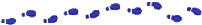## Expressions

Expressions perform the work of a Java program. Among other things, expressions are used to compute and assign values to variables and to help control the execution flow of a program. The job of an expression is two-fold: perform the computation indicated by the elements of the expression and return some value that is the result of the computation.

Definition: An expression is a series of variables, operators, and method calls (constructed according to the syntax of the language) that evaluates to a single value.

As discussed in the previous section, operators return a value, so the use of an operator is an expression. For example, the statement from the `countChars` method is an expression:

```count++;
```
This particular expression evaluates to the value of `count` before the operation occurs.

The data type of the value returned by an expression depends on the elements used in the expression. The expression `count++` returns an integer because `++` returns a value of the same data type as its operand and `count` is an integer. Other expressions return boolean values, strings, and so on.

The `countChars` method contains, in addition to the `count++` expression, a few other expressions, including this one:

```in.read() != -1
```
This expression is interesting because it consists of two expressions. The first expression is a method call:
```in.read()
```
A method call expression evaluates to the return value of the method; thus the data type of a method call expression is the same as the data type of the return value of that method. The `in.read` method is declared to return an integer so, the expression `in.read` evaluates to an integer.

The second expression contained in the statement `in.read() != -1` uses the `!=` operator. Recall that `!=` compares its two operands for inequality. In the statement in question the operands are `in.read` and `-1`. `in.read` is a valid operand for `!=` because `in.read` is an expression and evaluates to an integer. So `in.read() != -1` compares two integers, the value returned by `in.read` and `-1`. The value returned by `!=` is either `true` or `false` depending on the outcome of the comparison.

As you can see, Java allows you to construct compound expressions and statements from various smaller expressions as long as the data types required by one part of the expression matches the data types of the other. Also, as you may have concluded from the previous example, the order in which a compound expression is evaluated matters!

Take for example this compound expression:

```x * y * z
```
In this particular example, the order in which the expression is evaluated is unimportant because the results of multiplication is independent of order--the outcome is always the same no matter what order you apply the multiplications. However, this is not true of all expressions. For example, this expression gives different results depending on whether you perform the addition or the division operation first:
```x + y / 100
```
You can direct the Java compiler explicitly how you want an expression evaluated with balanced parentheses `(` and `)`. For example to make the previous expression unambiguous, you could write `(x + y)/ 100`.

If you don't explicitly tell the compiler the order in which you want operations to be performed, it decides based on the precedence assigned to the operators and other elements in use within the expression. Operators with a higher precedence get evaluated first. For example, the division operator has a higher precedence than does the addition operator so, in the compound expression shown previously, `x + y / 100`, the compiler would evaluate `y / 100` first. Thus

```x + y / 100
```
is equivalent to
```x + (y / 100)
```
To make your code easier to read and maintain you should be explicit and indicate with parentheses which operators should be evaluated first.

The following table shows the precedence assigned to Java's operators. The operators in this table are listed in precedence order: the higher in the table an operator appears, the higher its precedence. Operators with higher precedence are evaluated before operators with a relatively lower precedence. Operators on the same line have equal precedence.

 postfix operators `[] . (params) expr++ expr--` unary operators ` ++expr --expr +expr -expr ~ !` creation or cast `new (type)expr` multiplicative `* / %` additive `+ -` shift `<< >> >>>` relational `< > <= >= instanceof` equality `== !=` bitwise AND `&` bitwise exclusive OR `^` bitwise inclusive OR `|` logical AND `&&` logical OR `||` conditional `? :` assignment `= += -= *= /= %= &= ^= |= <<= >>= >>>=`

When operators of equal precendence appear in the same expression, some rule must govern which is evaluated first. In Java, all binary operators except for the assignment operators are evaluated in left to right order. Assignment operators are evaluated right to left.